# User Forum

Subject :IMO    Class : Class 5

State 'T' for true and 'F' for false.
(i) The fraction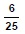is equal to the decimal number 0.24.
(ii) In proper fraction, numerator is smaller than the denominator.
(iii)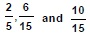are equivalent fractions.

A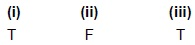B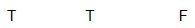C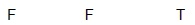D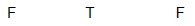there is no correct answer in the given optionsit is True, True, False.6/25 = 0.24 and in a proper fraction the numerator is smaller than denominator2/5, 6/15 are equivalent fractions but 10/15 is not.... so it is false (if it is given as 10/25 then it is true)

Class : Class 7